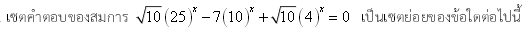# This seems familiar.What is the sum of all the real roots of the equation

$(\sqrt{10})25^{x}-7(10)^{x} + (\sqrt{10})4^{x} = 0$?

(I did not come up with this question. This is also from the entrance exam of the Computer POSN camp in Thailand.)

×